# Maths invention.ppt

28 May 2023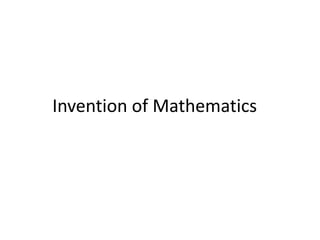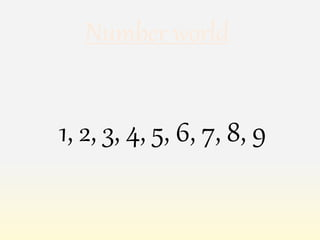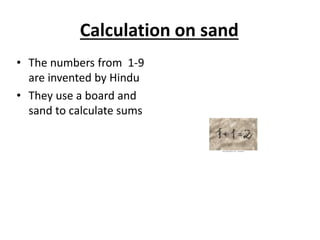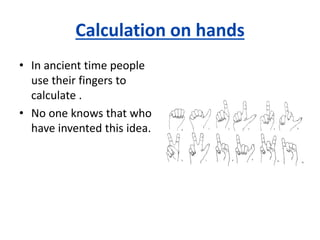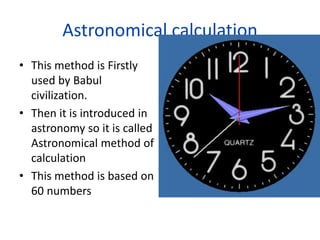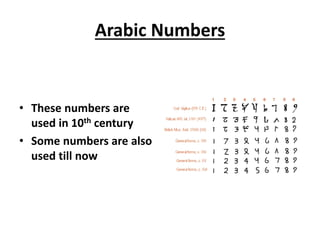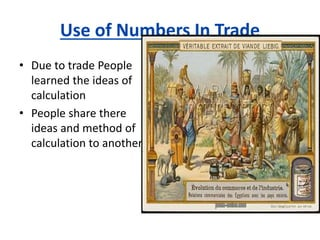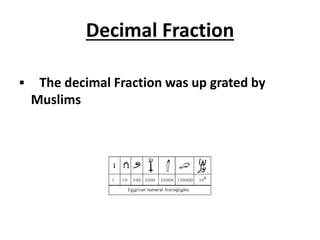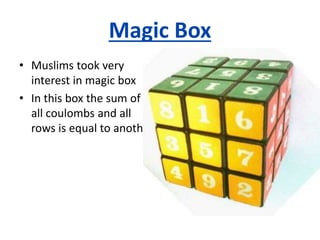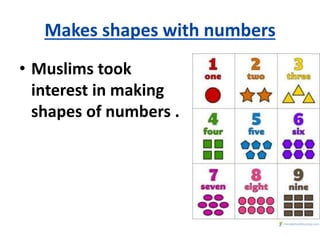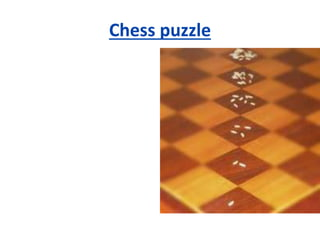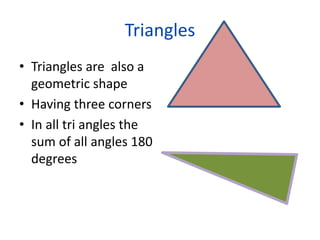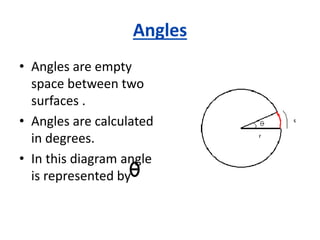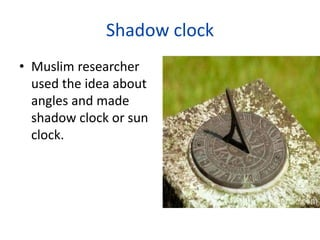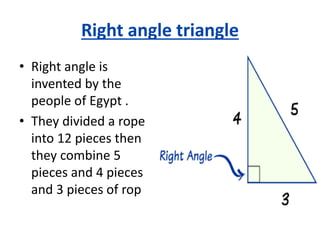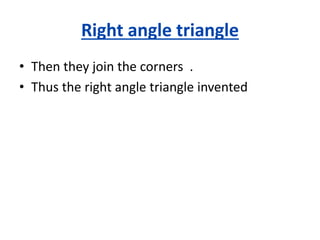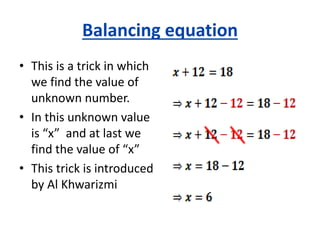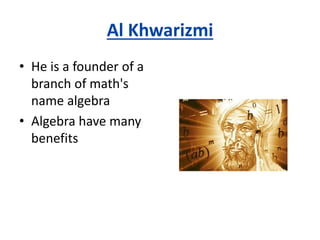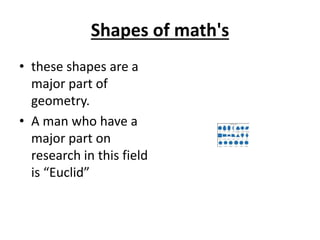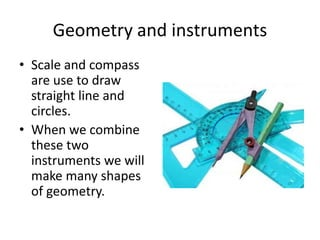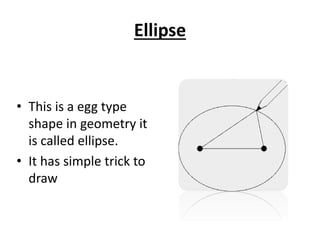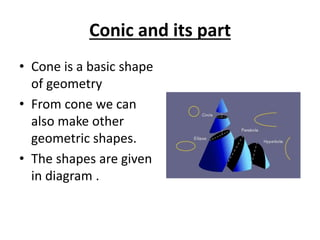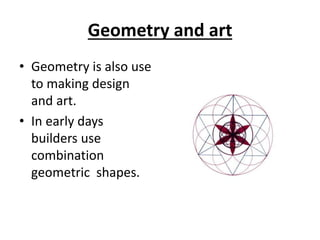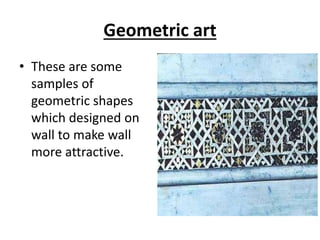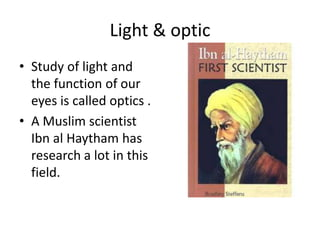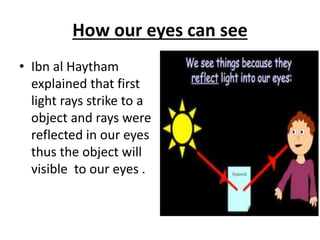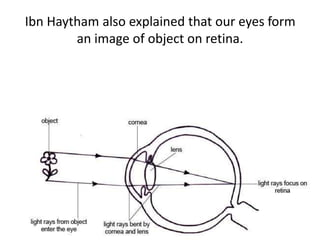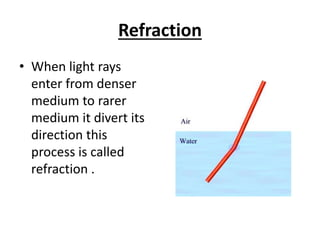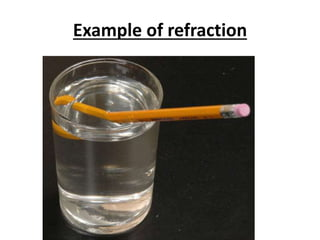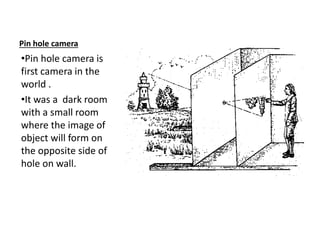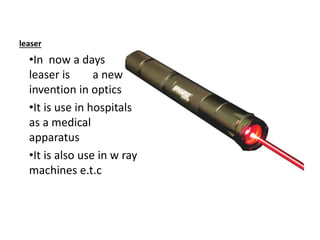1 sur 32

### Maths invention.ppt

• 1. Invention of Mathematics
• 2. 1, 2, 3, 4, 5, 6, 7, 8, 9 Number world
• 3. Calculation on sand • The numbers from 1-9 are invented by Hindu • They use a board and sand to calculate sums
• 4. Calculation on hands • In ancient time people use their fingers to calculate . • No one knows that who have invented this idea.
• 5. Astronomical calculation • This method is Firstly used by Babul civilization. • Then it is introduced in astronomy so it is called Astronomical method of calculation • This method is based on 60 numbers
• 6. Arabic Numbers • These numbers are used in 10th century • Some numbers are also used till now
• 7. Use of Numbers In Trade • Due to trade People learned the ideas of calculation • People share there ideas and method of calculation to anothers.
• 8. Decimal Fraction  The decimal Fraction was up grated by Muslims
• 9. Magic Box • Muslims took very interest in magic box • In this box the sum of all coulombs and all rows is equal to another
• 10. Makes shapes with numbers • Muslims took interest in making shapes of numbers .
• 11. Chess puzzle
• 12. Triangles • Triangles are also a geometric shape • Having three corners • In all tri angles the sum of all angles 180 degrees
• 13. Angles • Angles are empty space between two surfaces . • Angles are calculated in degrees. • In this diagram angle is represented by
• 15. Right angle triangle • Right angle is invented by the people of Egypt . • They divided a rope into 12 pieces then they combine 5 pieces and 4 pieces and 3 pieces of rop
• 16. Right angle triangle • Then they join the corners . • Thus the right angle triangle invented
• 17. Balancing equation • This is a trick in which we find the value of unknown number. • In this unknown value is “x” and at last we find the value of “x” • This trick is introduced by Al Khwarizmi
• 18. Al Khwarizmi • He is a founder of a branch of math's name algebra • Algebra have many benefits
• 19. Shapes of math's • these shapes are a major part of geometry. • A man who have a major part on research in this field is “Euclid”
• 20. Geometry and instruments • Scale and compass are use to draw straight line and circles. • When we combine these two instruments we will make many shapes of geometry.
• 21. Ellipse • This is a egg type shape in geometry it is called ellipse. • It has simple trick to draw
• 22. Conic and its part • Cone is a basic shape of geometry • From cone we can also make other geometric shapes. • The shapes are given in diagram .
• 23. Geometry and art • Geometry is also use to making design and art. • In early days builders use combination geometric shapes.
• 24. Geometric art • These are some samples of geometric shapes which designed on wall to make wall more attractive.
• 25. Light & optic • Study of light and the function of our eyes is called optics . • A Muslim scientist Ibn al Haytham has research a lot in this field.
• 26. How our eyes can see • Ibn al Haytham explained that first light rays strike to a object and rays were reflected in our eyes thus the object will visible to our eyes .
• 27. Ibn Haytham also explained that our eyes form an image of object on retina.
• 28. Refraction • When light rays enter from denser medium to rarer medium it divert its direction this process is called refraction .
• 29. Example of refraction
• 30. Pin hole camera •Pin hole camera is first camera in the world . •It was a dark room with a small room where the image of object will form on the opposite side of hole on wall.
• 31. leaser •In now a days leaser is a new invention in optics •It is use in hospitals as a medical apparatus •It is also use in w ray machines e.t.c